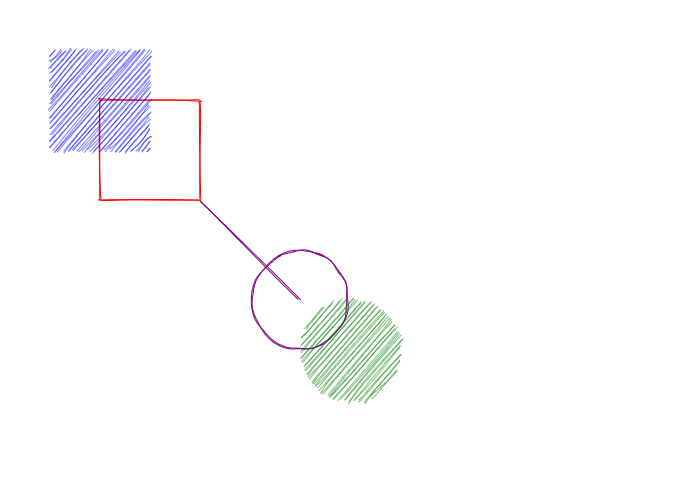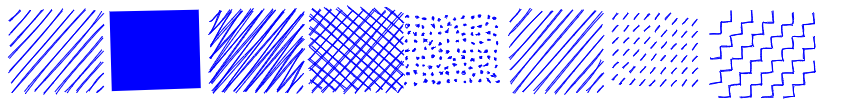# Rough Canvas#

ipycanvas provides a special canvas class that automatically gives a hand-drawn style to your drawings. The `RoughCanvas` API is the same as the `Canvas` one, except that it provides a few more options.

## Create a RoughCanvas#

You create a `RoughCanvas` the same way you would create a normal `Canvas`.

```from ipycanvas import RoughCanvas

canvas = RoughCanvas(width=200, height=200)
canvas
```

Then your drawings will have an hand-drawn style:

```from ipycanvas import RoughCanvas

canvas = RoughCanvas()

canvas.stroke_style = "red"
canvas.fill_style = "blue"
canvas.stroke_rect(100, 100, 100, 100)
canvas.fill_rect(50, 50, 100, 100)

canvas.stroke_style = "purple"
canvas.fill_style = "green"
canvas.stroke_circle(300, 300, 100)
canvas.fill_circle(350, 350, 100)

canvas.stroke_line(200, 200, 300, 300)

canvas
```Note

The `RoughCanvas` does not support yet applying the hand-drawn style to custom path commands (`begin_path`, `arc_to`, etc)

## Fill style#

The `RoughCanvas` provides a second fill style option `rough_fill_style` which changes the way shapes are filled.

```from ipycanvas import RoughCanvas

canvas = RoughCanvas(width=850, height=100)

canvas.fill_style = "blue"
canvas.line_width = 2.0

rough_fill_style_values = [
"hachure",
"solid",
"zigzag",
"cross-hatch",
"dots",
"sunburst",
"dashed",
"zigzag-line",
]

for i in range(len(rough_fill_style_values)):
canvas.rough_fill_style = rough_fill_style_values[i]

canvas.fill_rect(10 + i * 100, 10, 90, 80)

canvas
```## Roughness#

You can change the roughness of your drawing changing the `roughness` attribute (float). A rectangle with the roughness of 0 would be a perfect rectangle. There is no upper limit to this value, but a value over 10 is mostly useless.

```from ipycanvas import RoughCanvas

canvas = RoughCanvas(width=850, height=100)

canvas.fill_style = "green"

for i in range(8):
canvas.roughness = i

canvas.fill_rect(10 + i * 100, 10, 90, 80)

canvas
```## Bowing#

You can change the curves of your drawing changing the `bowing` attribute (float). It’s a numerical value indicating how curvy the lines are when drawing a sketch. A value of 0 will cause straight lines.

```from ipycanvas import RoughCanvas

canvas = RoughCanvas(width=850, height=150)

canvas.fill_style = "green"

for i in range(8):
canvas.bowing = i * 3.0

canvas.stroke_rect(20 + i * 100, 20, 90, 110)

canvas
```